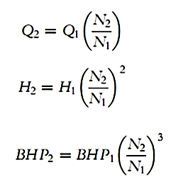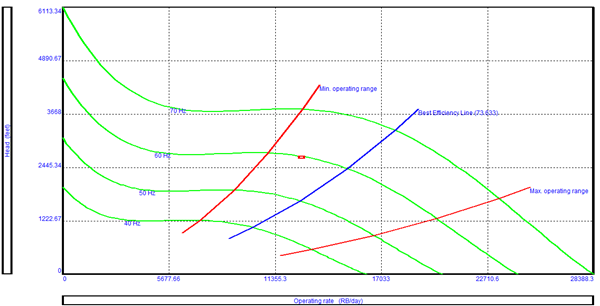# Affinity Laws

The affinity laws, also known as “Pump Laws”, for pumps are used to express the relationship between variables involved in pump performance (such as head, flow rate, shaft speed) and power.

According to the affinity laws, the following relationships exist between the actual speed of the centrifugal pump and its most important performance parameters:

• The flow rate of a pump changes directly proportional to its operating speed.
• The head developed by the pump changes proportionally to the square of the speed.
• The brake horsepower required to drive the pump changes proportionally to the cube of the speed.
• The efficiency of the pump does not change with speed changes.

The above rules can be expressed by the following equations:• N1 and N2 are pumping speeds (in RPM).
• Q1 and Q2 are pumping rates at pumping speeds N1 and N2 (in bpd).
• H1 and H2 are the developed heads at pumping speeds N1 and N2 (in ft).
• BHP1 and BHP2 are the required brake horsepower at pumping speeds N1 and N2 (in hp).

How Affinity Laws can be used to design the pump?

The ESP system can be modified to include a Variable Speed Drive (VSD); therefore it operates over a broader range of flow rate, head and efficiency. The VSD generates frequency goes from 30 to 90 Hz. Changing the operating frequency will change the motor speed, the pumping speed will be changed, so that the developed head and the required brake horsepower will change. Thus pump performance curves for frequencies other than 60 Hz (or 50 Hz) can be generated with the affinity laws.Each curve represents a series of points derived from the 60 Hz curve for flow and corresponding head points, transformed using the Affinity Law Equations.